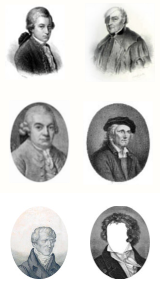# How it works

To play the 18th century dice game, the following accessories were required:

• Two dice;
• The Tables sheet with the measure indices;
• The Measures sheet with all the numbered bars;
• Music paper to write down your composition.
 I II III IV 2 96 22 141 41 3 32 6 128 63 4 69 95 158 13
Fig 1. Example indices table
The 'Measures' sheet consists of a large number of measures. These are not to be mistaken for a composition, the entire sheet is only a collection of measures. Of primary importance is the exact position of each measure on that sheet, which is linked to the 'Tables' sheet.

The 'Tables' sheet contains one or more tables containing indices linked to measures on the 'Measures' sheet (see figure 1 for an example of such a table).

The Roman numerals at the top of the eight columns of the Tables show the eight bars of the first (or second) part of the composition (either a minuet, a trio, or a waltz). The Arabic numerals preceding the rows, show the numbers which would result from throwing one or two dice. A Roman and an Arabic numeral together specify the measure to be selected in the 'Measures' section.

Please note that working with indices is a bit superfluous. The measures could also have been entered directly in the Table, which would save the tedious work of looking them up by their number. We have chosen to leave this aspect of the game intact.

## Playing the (original) game

We will use figure 1 above to illustrate how the game is played. With that table it is possible to create compositions of four bars length, with three variations for each position. So, in total, we can generate 12 different pieces with this table.

The game begins. If the first roll of the dice produces e.g. a '4', then the measure number in the first column would be '69'. That would be the first bar of our composition. Now, if rolling the dice for the second bar would produce a '3', then the second bar number as found in column II of the Table would be '6', etc. We now have two indices, '69' and '6' (in that order). The last step is to look up the corresponding measures in the list of measures and create a composition. In this application we use the computer to do all the dice work. Don't worry, it knows what it is doing.

Using the computer means that we no longer depend on the results of emulating the throwing of dice. Using some clever techniques it is possible to guide the computer in its choice of measures. In the application we did this by letting the user input names and birth dates to generate unique compositions. The 'Composition options' button, top left on the 'Compose' page, presents five possible ways to 'compose' your music. Exactly how this is done is explained on the 'Discussion' page.